CBSE Class 12 Sample Paper for 2019 Boards

Class 12
Solutions of Sample Papers and Past Year Papers - for Class 12 Boards

Question 21 (OR 1 st question)

Find the particular solution of the following differential equation.

cos y dx + (1 + 2e -x ) sin y dy = 0; y(0) = π/4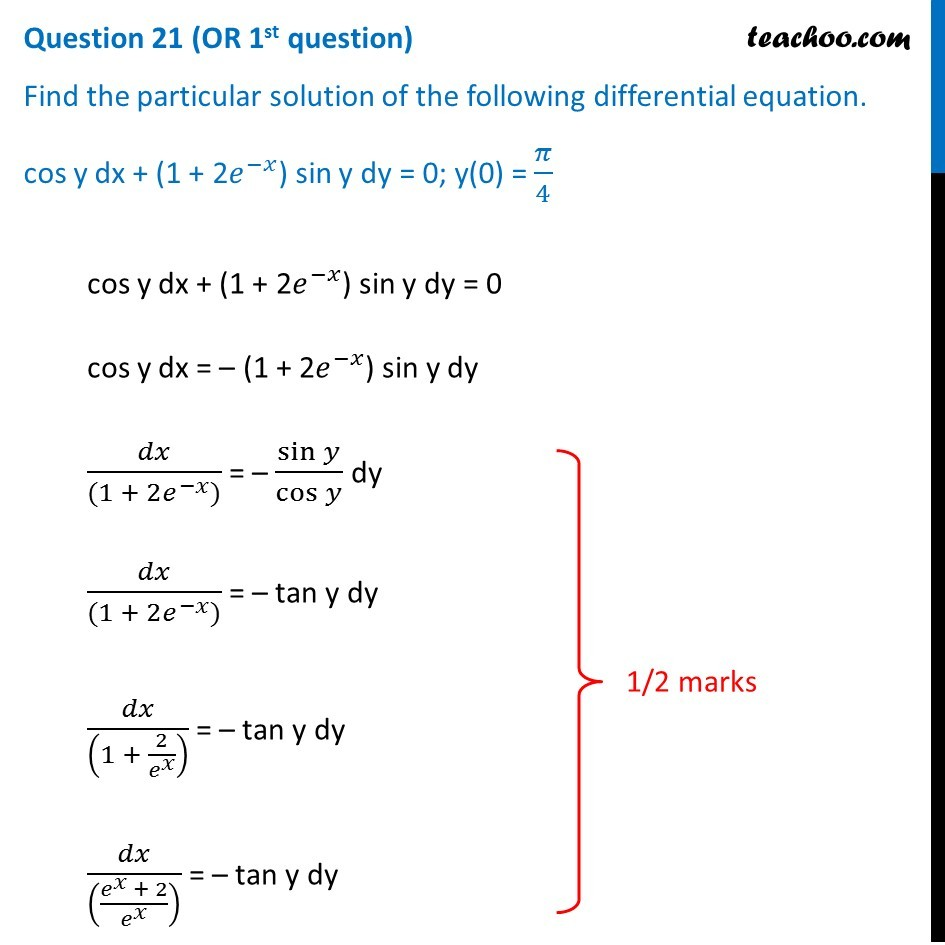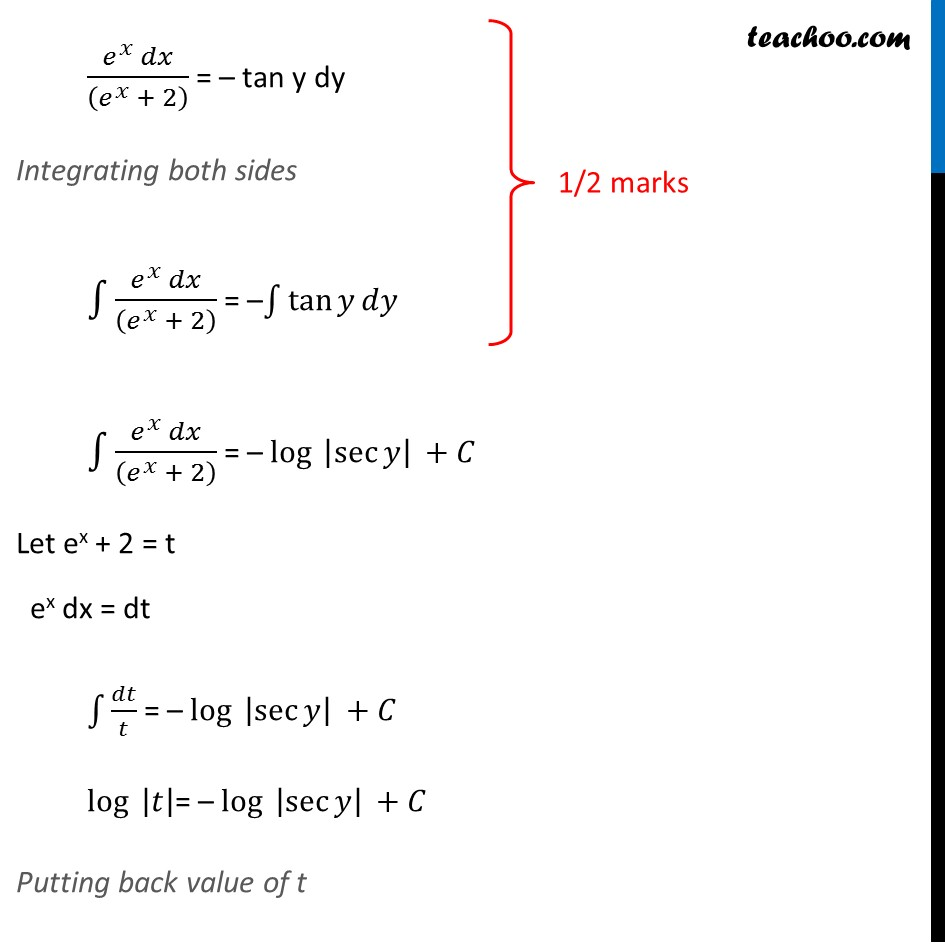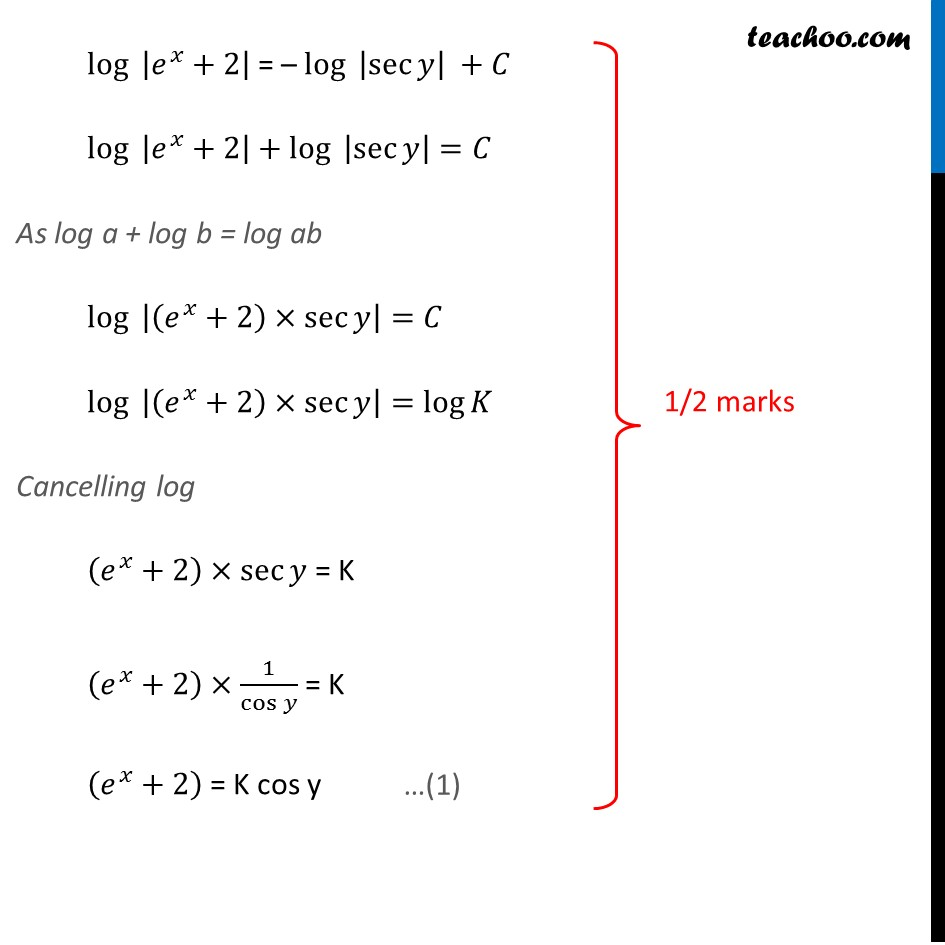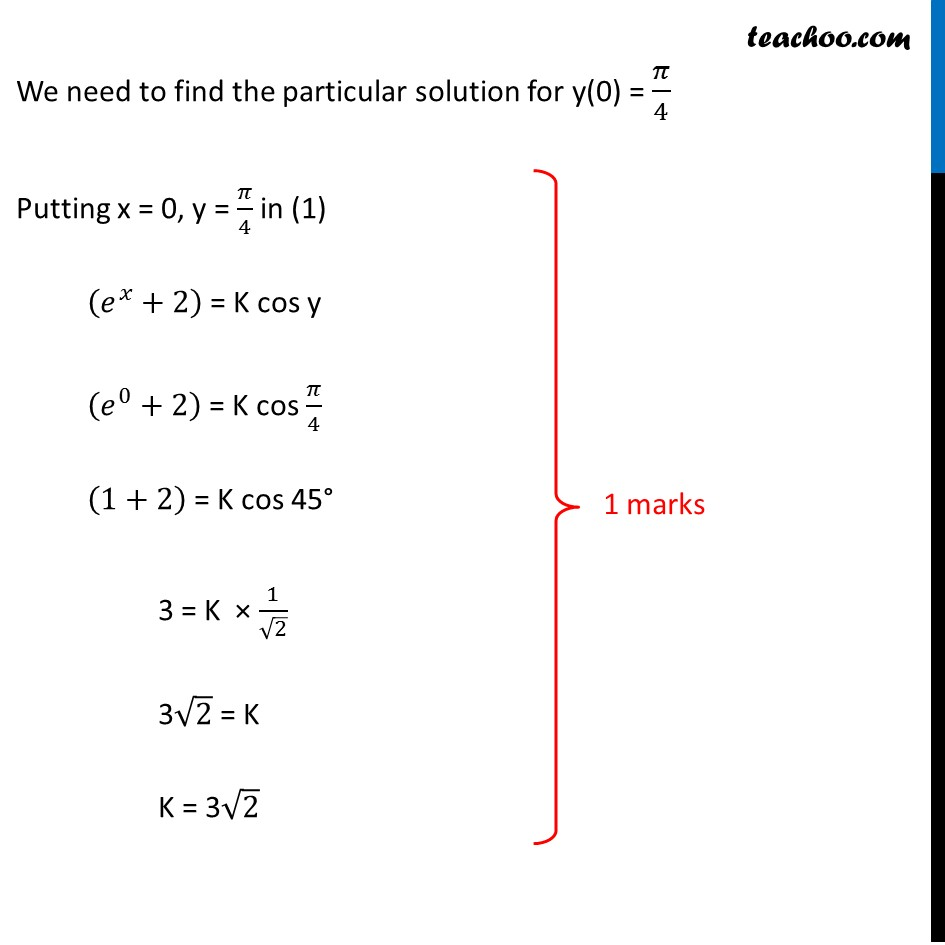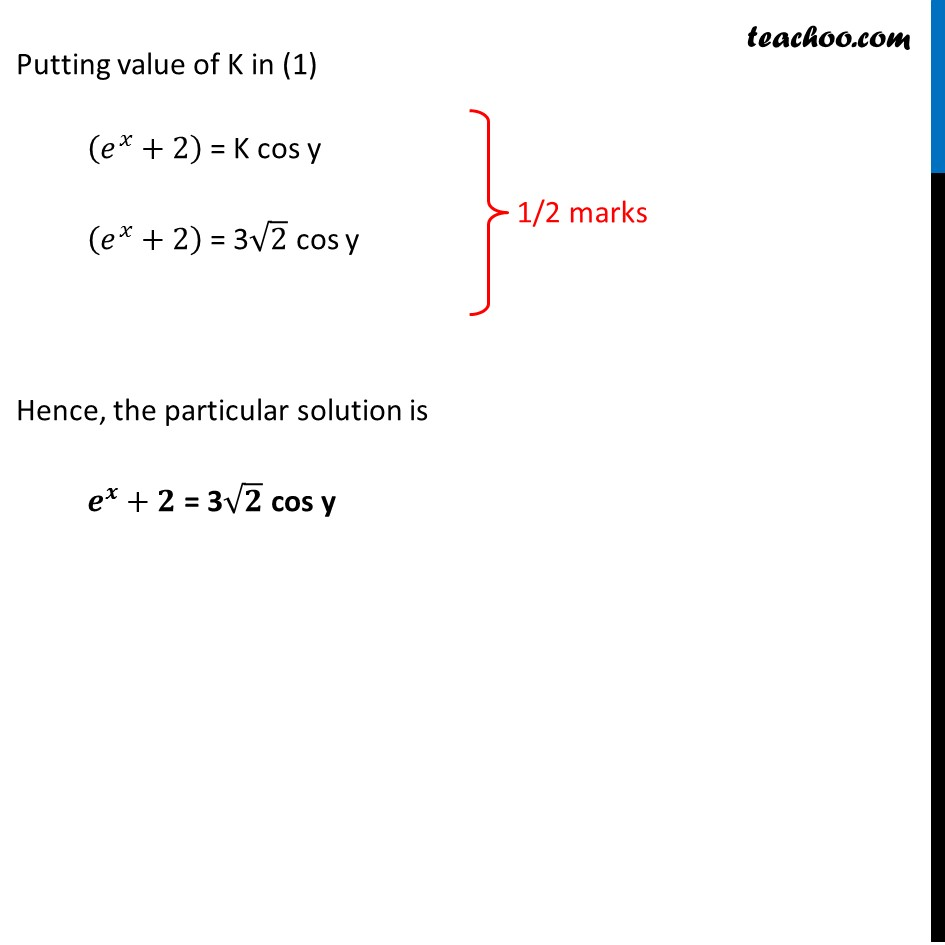Learn in your speed, with individual attention - Teachoo Maths 1-on-1 Class

### Transcript

Question 21 (OR 1st question) Find the particular solution of the following differential equation. cos y dx + (1 + 2𝑒^(−𝑥)) sin y dy = 0; y(0) = 𝜋/4 cos y dx + (1 + 2𝑒^(−𝑥)) sin y dy = 0 cos y dx = – (1 + 2𝑒^(−𝑥)) sin y dy 𝑑𝑥/((1 + 〖2𝑒〗^(−𝑥))) = – sin⁡𝑦/cos⁡𝑦 dy 𝑑𝑥/((1 + 〖2𝑒〗^(−𝑥))) = – tan y dy 𝑑𝑥/((1 + 2/𝑒^𝑥 ) ) = – tan y dy 𝑑𝑥/(((𝑒^𝑥 + 2)/𝑒^𝑥 ) ) = – tan y dy (𝑒^𝑥 𝑑𝑥)/((𝑒^𝑥 + 2) ) = – tan y dy Integrating both sides ∫1▒(𝑒^𝑥 𝑑𝑥)/((𝑒^𝑥 + 2) ) = –∫1▒〖tan⁡𝑦 𝑑𝑦〗 ∫1▒(𝑒^𝑥 𝑑𝑥)/((𝑒^𝑥 + 2) ) = – log⁡〖 |sec⁡𝑦 |〗 +𝐶 Let ex + 2 = t ex dx = dt ∫1▒𝑑𝑡/𝑡 = – log⁡〖 |sec⁡𝑦 |〗 +𝐶 log⁡〖 |𝑡|〗= – log⁡〖 |sec⁡𝑦 |〗 +𝐶 Putting back value of t log⁡〖 |𝑒^𝑥+2|〗 = – log⁡〖 |sec⁡𝑦 |〗 +𝐶 log⁡〖 |𝑒^𝑥+2|〗+ log⁡〖 |sec⁡𝑦 |〗=𝐶 As log a + log b = log ab log⁡〖 |(𝑒^𝑥+2)×sec⁡𝑦 |〗=𝐶 log⁡〖 |(𝑒^𝑥+2)×sec⁡𝑦 |〗=log⁡𝐾 Cancelling log (𝑒^𝑥+2)×sec⁡𝑦 = K (𝑒^𝑥+2)×1/cos⁡𝑦 = K (𝑒^𝑥+2) = K cos y log⁡〖 |𝑒^𝑥+2|〗 = – log⁡〖 |sec⁡𝑦 |〗 +𝐶 log⁡〖 |𝑒^𝑥+2|〗+ log⁡〖 |sec⁡𝑦 |〗=𝐶 As log a + log b = log ab log⁡〖 |(𝑒^𝑥+2)×sec⁡𝑦 |〗=𝐶 log⁡〖 |(𝑒^𝑥+2)×sec⁡𝑦 |〗=log⁡𝐾 Cancelling log (𝑒^𝑥+2)×sec⁡𝑦 = K (𝑒^𝑥+2)×1/cos⁡𝑦 = K (𝑒^𝑥+2) = K cos y We need to find the particular solution for y(0) = 𝜋/4 Putting x = 0, y = 𝜋/4 in (1) (𝑒^𝑥+2) = K cos y (𝑒^0+2) = K cos 𝜋/4 (1+2) = K cos 45° 3 = K × 1/√2 3√2 = K K = 3√2 Putting value of K in (1) (𝑒^𝑥+2) = K cos y (𝑒^𝑥+2) = 3√2 cos y Hence, the particular solution is 𝒆^𝒙+𝟐 = 3√𝟐 cos y